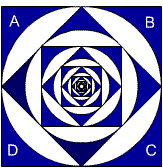#### You may also like### Polycircles

Show that for any triangle it is always possible to construct 3 touching circles with centres at the vertices. Is it possible to construct touching circles centred at the vertices of any polygon?### Nim

Start with any number of counters in any number of piles. 2 players take it in turns to remove any number of counters from a single pile. The loser is the player who takes the last counter.### Loopy

Investigate sequences given by $a_n = \frac{1+a_{n-1}}{a_{n-2}}$ for different choices of the first two terms. Make a conjecture about the behaviour of these sequences. Can you prove your conjecture?

# Squaring the Circle and Circling the Square

##### Age 14 to 16Challenge Level
 Part 1 1) What is the area of the red portion of this figure? Click NEXT 2) What is the area of the blue portion of this figure? Click NEXT 3) What is the area of the orange portion of this figure? If you continue the pattern, can you predict what each of the following areas will be? Try to explain your prediction.
 Part 2 Now imagine that instead of the pattern growing, we start with a square and the pattern continues inwards - with the circles and squares becoming smaller and smaller. If the areas of the four blue shapes labelled A, B, C and D are one unit each, what is the combined area of all the blue shapes? Explain any reasoning you have used.